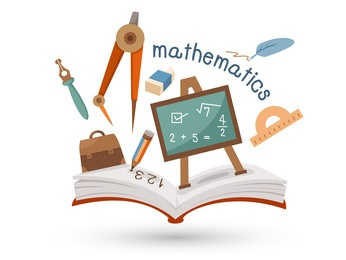# Fifth Grade : Free Math Worksheets

Find more about prime and composite numbers, organized lists, and number theory. There is another level of decimals and fractions and their usage in algebraic expressions and equations. Get to know about percentages, accounting, and interest calculation. Master perimeter, area, and volume. Learn about ordered pairs, linear functions, and graphing of simple equations.

Here is the list of all the topics that students learn in this grade. There are some sample worksheets below each section to provide a sense of what to expect. Each section has some free worksheets too. Please subscribe to access the whole content in its best form.We are aligned to common core standards. Click the button below to the see the curriculum.
Common Core

Click on any topic to view, print, or download the worksheets. All of our worksheets are free for non-commercial and personal use.

Featured Content

Reading Graphs and Reasoning - I

Reading data from double bar graphs and pie charts to solve problems based on interpretation of the data. Explain the use cases for line graphs.

Category:  Data and Graphs     Data Analysis     Reading Graphs and Reasoning